ICSE Class 10 Heights and Distances Solution New Pattern

## ICSE Class 10 Heights and Distances Solution By Clarify Knowledge

ICSE Class 10 Heights and Distances Solution New Pattern 2022

## Chapter 21 - Heights and Distances Ex. 21(A)

Question 1

The height of a tree is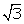times the length of its shadow. Find the angle of elevation of the sun.Solution 1

Let the length of the shadow of the tree be x m.Height of the tree =m

Ifis the angle of elevation of the sun, then

Question 2

The angle of elevation of the top of a tower from a point on the ground and at a distance of 160 m from its foot, is found to be 60o. Find the height of the tower.Solution 2

Let the height of the tower be h m.

Given that angle of elevation is 60o

So, height of the tower is 277.12 m.Question 3

A ladder is placed along a wall such that its upper end is resting against a vertical wall. The foot of the ladder is 2.4 m from the wall and the ladder is making an angle of 68o with the ground. Find the height, upto which the ladder reaches.Solution 3

Let the height upto which the ladder reaches be h m.

Given that angle of elevation is 68o

So, the ladder reaches upto a height of 5.94 m.Question 4

Two persons are standing on the opposite sides of a tower. They observe the angles of elevation of the top of the tower to be 30o and 38o respectively. Find the distance between them, if the height of the tower is 50 m.Solution 4

Let one person A be at a distance x and the second person B be at a distance of y from the foot of the tower.

Given that angle of elevation of A is 30o

The angle of elevation of B is 38o

So, distance between A and B is x + y = 150.6 mQuestion 5

A kite is attached to a string. Find the length of the string, when the height of the kite is 60 m and the string makes an angle 30o with the ground.Solution 5

Let the length of the rope be x m.

Now,

So, the length of the rope is 120m.Question 6

A boy, 1.6 m tall, is 20 m away from a tower and observes the angle of elevation of the top of the tower to be (i) 45o, (ii) 60o. Find the height of the tower in each case.Solution 6

Let the height of the tower be h m.

(i) Here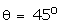So, height of the tower is 21.6 m.

(ii) HereSo, height of the tower is 36.24 m.Question 7

The upper part of a tree, broken over by the wind, makes an angle of 45o with the ground and the distance from the root to the point where the top of the tree touches the ground is 15 m. What was the height of the tree before it was broken?Solution 7

Let the height of the tree after breaking be h m.

HereNow, length of the tree broken by the wind =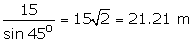So, height of the tree before it was broken is (15 + 21.21) m = 36.21 m.Question 8

The angle of elevation of the top of an unfinished tower at a point distance 80 m from its base is 30o. How much higher must the tower be raised so that its angle of elevation at the same point may be 60o?Solution 8

Let AB be the unfinished tower and C be the top of the tower when finished. Let P be a point 80 m from the foot A.

InBAP,

InCAP,

Therefore, the tower must be raised by (138.56 - 46.19)m = 92.37 mQuestion 9

At a particular time, when the sun's altitude is 30o, the length of the shadow of a vertical tower is 45 m. Calculate

(i) the length of the tower.

(ii) the length of the shadow of the same tower, when the sun's altitude is

(a) 45o (b) 60oSolution 9

Let the length of the tower be h m.

(i) Here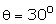Hence the length of the tower is 25.98 m.

(ii) Let the length of the shadow be x m.

(a) Here,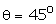Hence the length of the shadow is 25.98 m

(b) Here,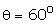Hence the length of the shadow is 15 m.Question 10

Two vertical poles are on either side of a road. A 30 m long ladder is placed between the two poles. When the ladder rests against one pole, it makes angle 32o24' with the pole and when it is turned to rest against another pole, it makes angle 32o24' with the road. Calculate the width of the road.Solution 10

Let AB be the ladder andABP = 32o24'.

When rotated, let the ladder be AC andCAQ = 32o24'.

Hence, width of the road = (16.08 + 25.32) = 41.4 mQuestion 11

Two climbers are at points A and B on a vertical cliff face. To an observer C, 40m from the foot of the cliff, on the level ground, A is at an elevation of 48o and B of 57o. What is the distance between the climbers?Solution 11

Let P be the foot of the cliff on level ground.

Then,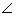ACP = 48o andBCP = 57o

Hence, distance between the climbers = AB = BP - AP = 17.16 mQuestion 12

A man stands 9 m away from a flag-pole. He observes that angle of elevation of the top of the pole is 28o and the angle of depression of the bottom of the pole is 13o. Calculate the height of the pole.Solution 12

Let AB be the man and PQ be the flag-pole.

Given, AR = 9 m.

Also,PAR = 28o andQAR = 13o

Hence, height of the pole = PR + RQ = 6.867 mQuestion 13

From the top of a cliff 92 m high, the angle of depression of a buoy is 20o. Calculate, to the nearest metre, the distance of the buoy from the foot of the cliff.Solution 13

Let AB be the cliff and C be the buoy.

Given, AB = 92 m.

Also,ACB = 20o

Hence, the buoy is at a distance of 253 m from the foot of the cliff.

## Chapter 22 - Heights and Distances Exercise Ex. 22(B)

Question 1

In the figure, given below, it is given that AB is perpendicular to BD and is of length X metres. DC = 30 m,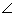ADB = 30o andACB = 45o. Without using tables, find X.

Solution 1

Question 2

Find the height of a tree when it is found that on walking away from it 20 m, in a horizontal line through its base, the elevation of its top changes from 60o to 30o.Solution 2

Let AB be the tree of height h m.

Let the two points be C and D such that CD = 20 m,ADB = 30o andACB = 60o

Hence, height of the tree is 17.32 m.Question 3

Find the height of a building, when it is found that on walking towards it 40 m in a horizontal line through its base the angular elevation of its top changes from 30o to 45o.Solution 3

Let AB be the building of height h m.

Let the two points be C and D such that CD = 40 m,ADB = 30o andACB = 45o

Hence, height of the building is 54.64 m.Question 4

From the top of a light house 100 m high, the angles of depression of two ships are observed as 48o and 36o respectively. Find the distance between the two ships(in the nearest metre) if:

(i) the ships are on the same side of the light house.

(ii) the ships are on the opposite sides of the light house.Solution 4

Let AB be the lighthouse.

Let the two ships be C and D such thatADB = 36o andACB = 48o

(i) If the ships are on the same side of the light house,

then distance between the two ships = BD - BC = 48 m

(ii) If the ships are on the opposite sides of the light house,

then distance between the two ships = BD + BC = 228 mQuestion 5

Two pillars of equal heights stand on either side of a roadway, which is 150 m wide. At a point in the roadway between the pillars the elevations of the tops of the pillars are 60o and 30o ; find the height of the pillars and the position of the point.Solution 5

Let AB and CD be the two towers of height h m.

Let P be a point in the roadway BD such that BD = 150 m,APB = 60o andCPD = 30o

Hence, height of the pillars is 64.95 m.

The point is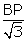from the first pillar.

That is the position of the point is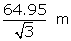from the first pillar.

The position of the point is 37.5 m from the first pillar.Question 6

From the figure, given below, calculate the length of CD.

Solution 6

Question 7

The angle of elevation of the top of a tower is observed to be 60o. At a point, 30 m vertically above the first point of observation, the elevation is found to be 45o. Find:

(i) the height of the tower,

(ii) its horizontal distance from the points of observation.Solution 7

Let AB be the tower of height h m.

Let the two points be C and D such that CD = 30 m,ADE = 45o andACB = 60o

Hence, height of the tower is 70.98 m

(ii)

The horizontal distance from the points of observation is BC = 40.98 mQuestion 8

From the top of a cliff, 60 metres high, the angles of depression of the top and bottom of a tower are observed to be 30o and 60o. Find the height of the tower.Solution 8

Let AB be the cliff and CD be the tower.

Here AB = 60 m,ADE = 30o andACB = 60o

Hence, height of the tower is 40 m.Question 9

A man on a cliff observes a boat, at an angle of depression 30o, which is sailing towards the shore to the point immediately beneath him. Three minutes later, the angle of depression of the boat is found to be 60o. Assuming that the boat sails at a uniform speed, determine:

(i) how much more time it will take to reach the shore.

(ii) the speed of the boat in metre per second, if the height of the cliff is 500 m.Solution 9

Let AB be the cliff and C and D be the two positions of the boat such thatADE = 30o andACB = 60o

Let speed of the boat be x metre per minute and let the boat reach the shore after t minutes more.

Therefore, CD = 3x m ; BC = tx m

Hence, the boat takes an extra 1.5 minutes to reach the shore.

And, if the height of cliff is 500 m, the speed of the boat is 3.21 m/secQuestion 10

A man in a boat rowing away from a lighthouse 150 m high, takes 2 minutes to change the angle of elevation of the top of the lighthouse from 60o to 45o. Find the speed of the boat.Solution 10

Let AB be the lighthouse and C and D be the two positions of the boat such that AB = 150 m,ADB = 45o andACB = 60o

Let speed of the boat be x metre per minute.

Therefore, CD = 2x m ;

Hence, the speed of the boat is 0.53 m/secQuestion 11

A person standing on the bank of a river observes that the angle of elevation of the top of a tree standing on the opposite bank is 60o. When he moves 40 m away from the bank, he finds the angle of elevation to be 30o. Find:

(i) the height of the tree, correct to 2 decimal places,

(ii) the width of the river.Solution 11

Let AB be the tree of height 'h' m and BC be the width of the river. Let D be the point on the opposite bank of tree such that CD = 40 m. HereADB = 30o andACB = 60o

Let speed of the boat be x metre per minute.

Hence, height of the tree is 34.64 m and width of the river is 20 m.Question 12

The horizontal distance between two towers is 75 m and the angular depression of the top of the first tower as seen from the top of the second, which is 160 m high, is 45o. Find the height of the first tower.Solution 12

Let AB and CD be the two towers

The height of the first tower is AB = 160 m

The horizontal distance between the two towers is

BD = 75 m

And the angle of depression of the first tower as seen from the top of the second tower isACE = 45o.

Hence, height of the other tower is 85 mQuestion 13

The length of the shadow of a tower standing on level plane is found to be 2y metres longer when the sun's altitude is 30o than when it was 45o. Prove that the height of the tower ismetres.Solution 13

Let AB be the tower and C and D are two points such that CD = 2y m,ADB = 45o andACB = 30o

Hence, height of the tower ism.Question 14

An aeroplane flying horizontally 1 km above the ground and going away from the observer is observed at an elevation of 60o. After 10 seconds, its elevation is observed to be 30o; find the uniform speed of the aeroplane in km per hour.Solution 14

Let A be the aeroplane and B be the observer on the ground. The vertical height will be AC = 1 km = 1000 m. After 10 seconds, let the aeroplane be at point D.

Let the speed of the aeroplane be x m/sec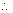CE = 10x

Hence, speed of the aeroplane is 415.69 km/hrQuestion 15

From the top of a hill, the angles of depression of two consecutive kilometer stones, due east, are found to be 30o and 45o respectively. Find the distances of the two stones from the foot of the hill.Solution 15

Let AB be the hill of height 'h' km and C and D be the two consecutive stones such that CD = 1 km,ACB = 30o andADB = 45o.

Hence, the two stones are at a distance of 1.366 km and 2.366 km from the foot of the hill.

## Chapter 22 - Heights and Distances Exercise Ex. 22(C)

Question 1

(i)

(ii)

Solution 1

Question 2

In the following diagram, AB is a floor-board; PQRS is a cubical box with each edge = 1 m andB = 60o. Calculate the length of the board AB.

Solution 2

Question 3

Calculate BC.

Solution 3

Question 4

Calculate AB.

Solution 4

Question 5

The radius of a circle is given as 15 cm and chord AB subtends an angle of 131o at the centre C of the circle. Using trigonometry, calculate:

(i) the length of AB;

(ii) the distance of AB from the centre C.Solution 5

Given, CA = CB = 15 cm,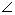ACB = 131o

Drop a perpendicular CP from centre C to the chord AB.

Then CP bisectsACB as well as chord AB.

(ii) CP = AC cos (65.5o)

=15×0.415 = 6.225 cm.Question 6

At a point on level ground, the angle of elevation of a vertical tower is found to be such that its tangent is. On walking 192 metres towards the tower, the tangent of the angle is found to be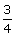. Find the height of the tower.Solution 6

Let AB be the vertical tower and C and D be two points such that CD = 192 m. LetACB =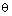andADB =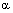.

Hence, the height of the tower is 180 m.Question 7

A vertical tower stands on a horizontal plane and is surmounted by a vertical flagstaff of height h metre. At a point on the plane, the angle of elevation of the bottom of the flagstaff isand at the top of the flagstaff is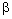. Prove that the height of the tower is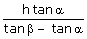.Solution 7

Let AB be the tower of height x metre, surmounted by a vertical flagstaff AD. Let C be a point on the plane such that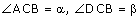and AD = h.

Question 8

With reference to the given figure, a man stands on the ground at point A, which is on the same horizontal plane as B, the foot of the vertical pole BC. The height of the pole is 10 m. The man's eye s 2 m above the ground. He observes the angle of elevation of C, the top of the pole, as xo , where tan xo =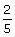. Calculate:

(i) the distance AB in metres;

(ii) angle of elevation of the top of the pole when he is standing 15 metres from the pole. Give your answer to the nearest degree.Solution 8

Let AD be the height of the man, AD = 2 m.

Question 9

The angles of elevation of the top of a tower from two points on the ground at distances a and b metres from the base of the tower and in the same line are complementary. Prove that the height of the tower ismetre.Solution 9

Let AB be the tower of height h metres.

Let C and D be two points on the level ground such that BC = b metres, BD = a metres,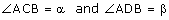.

Question 10

From a window A, 10 m above the ground the angle of elevation of the top C of a tower is xo, where tan xo =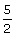and the angle of depression of the foot D of the tower is yo, where tan yo =. Calculate the height CD of the tower in metres.

Solution 10

Question 11

A vertical tower is 20 m high. A man standing at some distance from the tower knows that the cosine of the angle of elevation of the top of the tower is 0.53. How far is he standing from the foot of the tower?Solution 11

Let AB be the tower of height 20 m.

Letbe the angle of elevation of the top of the tower from point C.

Question 12

A man standing on the bank of a river observes that the angle of elevation of a tree on the opposite bank is 60o. When he moves 50 m away from the bank, he finds the angle of elevation to be 30o. Calculate:

(i) the width of the river;

(ii) the height of the tree.Solution 12

Let AB be the tree and AC be the width of the river. Let D be a point such that CD = 50 m. Given thatQuestion 13

A 20 m high vertical pole and a vertical tower are on the same level ground in such a way that the angle of elevation of the top of the tower, as seen from the foot of the pole is 60o and the angle of elevation of the top of the pole, as seen from the foot of the tower is 30o. Find:

(i) the height of the tower ;

(ii) the horizontal distance between the pole and the tower.Solution 13

Question 14

A vertical pole and a vertical tower are on the same level ground in such a way that from the top of the pole, the angle of elevation of the top of the tower is 60o and the angle of depression of the bottom of the tower is 30o. Find:

(i) the height of the tower, if the height of the pole is 20 m;

(ii) the height of the pole, if the height of the tower is 75 m.Solution 14

Let AB be the tower and CD be the pole.

Then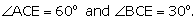Question 15

From a point, 36 m above the surface of a lake, the angle of elevation of a bird is observed to be 30o and the angle of depression of its image in the water of the lake is observed to be 60o. Find the actual height of the bird above the surface of the lake.Solution 15

Let A be a point 36 m above the surface of the lake and B be the position of the bird. Let B' be the image of the bird in the water.

Question 16

A man observes the angle of elevation of the top of a building to be 30o. He walks towards it in a horizontal line through its base. On covering 60 m, the angle of elevation changes to 60o. Find the height of the building correct to the nearest metre.Solution 16

Let AB be a building and M and N are the two positions of the man which makes angles of elevation of top of building as 30o and 60respectively.

MN = 60 m

Let AB = h and NB = x m

Question 17

As observed from the top of a 80 m tall lighthouse, the angles of depression of two ships, on the same side of a light house in a horizontal line with its base, are 30° and 40° respectively. Find the distance between the two ships. Give your answer corrected to the nearest metre.Solution 17

Let AB represent the lighthouse.

Let the two ships be at points D and C having angle of depression 30° and 40° respectively.

Let x be the distance between the two ships.

The distance between the two ships is 43 m.Question 18

In the given figure, from the top of a building AB = 60 m high, the angles of depression of the top and bottom of a vertical lamp post CD are observed to be 30° and 60° respectively. Find :

(i) the horizontal distance between AB and CD.

(ii) the height of the lamp post.

Solution 18

Question 19

An aeroplane, at an altitude of 250 m, observes the angles of depression of two boats on the opposite banks of a river to be 45° and 60° respectively. Find the width of the river. Write the answer correct to the nearest whole number.Solution 19

Let A be the position of the airplane and let BC be the river. Let D be the point in BC just below the airplane.

B and C be two boats on the opposite banks of the river with angles of depression 60° and 45° from A.

Question 20

The horizontal distance between two towers is 120 m. The angle of elevation of the top and angle of depression of the bottom of the first tower as observed from the top of the second tower is 30° and 24° respectively. Find the height of the two towers. Give your answers. Give your answer correct to 3 significant figures.

Solution 20

Question 21

The angles of depression of two ships A and B as observed from the top of a light house 60m high, are 60° and 45° respectively. If the two ships are on the opposite sides of the light house, find the distance between the two ships. Give your answer correct to the nearest whole number.Solution 21

In the above figure

OT=tower = 60m

A and B are the respective positions of ship

error: Content is protected !!